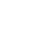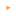Simcountry is an Online Digital World where you are the President of a country.No download needed!What is Simcountry? Beginners InfoTweetFriday, January 02, 2004 - 04:16 pmHi all. I just registered a new country and was looking around it and came across something I haven't seen before (I use to play a country a while back). Under the fincial index, what in the world is Material Accounting.... What ever it is it's currently running an almost bigger deficit then my country has assets and is losing 50 billion a month. Friday, January 02, 2004 - 09:14 pmQuick and dirty... The material accounting is what covers the goods your people buy from the government. It is a cash account used to buy and sell goods that your people consume. It has always been there, but W3C just recently added the section on the finance page that shows us what it is doing. I believe it also covers the "Strategic" goods that you sell to corporations. Keith Saturday, January 03, 2004 - 10:31 pmSo does it have an effect on anything: score, economy, or welfare? And if so... how do I balance it? Sunday, January 04, 2004 - 01:44 amSome of the cost is added to the general cost of government, last I looked I was getting 4B tacked on per month. Wednesday, January 14, 2004 - 10:22 pmHi me again. Now I have a question about GR weapon ranges. Defensive short-range missile batteries have a range of 800 miles. Cruise missile batteries have a range of 3000 miles. Does this mean I can strike with impunity at a target deffend by only SRMBs at a range of 800 to 3000 miles? Also how long is a mile.... Thursday, January 15, 2004 - 12:30 amNautical (for your Navy) 1 (nautical) mile = 6,076.1154856 feet In Meters> 1 (nautical) mile = 1,852 meters Statute (for your Army) 1 (statute) mile = 5,280.01056 feet In Meters> 1 (statute) mile = 1,609.3472187 meters Friday, January 16, 2004 - 01:28 amYes... but how far is that in latitude and logitude As in how can I tell how far away a target is. Friday, January 16, 2004 - 03:33 amLatitude and Longitude, system of geometrical coordinates used in designating the location of places on the surface of the earth. (For the use of these terms in astronomy, see Coordinate System; Ecliptic.) Latitude, which gives the location of a place north or south of the equator, is expressed by angular measurements ranging from 0° at the equator to 90° at the poles. Longitude, the location of a place east or west of a north-south line called the prime meridian, is measured in angles ranging from 0° at the prime meridian to 180° at the International Date Line. Midway between the poles, the equator, a great circle, divides the earth into northern and southern hemispheres. Parallel to the equator and north and south of it are a succession of imaginary circles that become smaller and smaller the closer they are to the poles. This series of east-west-running circles, known as the parallels of latitude, is crossed at right angles by a series of half-circles extending north and south from one pole to the other, called the meridians of longitude. Although the equator was an obvious choice as the prime parallel, being the largest, no one meridian was uniquely qualified as prime. Until a single prime meridian could be agreed upon, each nation was free to choose its own, with the result that many 19th-century maps of the world lacked a standardized grid. The problem was resolved in 1884, when an international prime meridian, passing through London's Greenwich Observatory, was officially designated. A metallic marker there indicates its exact location. Degrees of latitude are equally spaced, but the slight flattening at the poles causes the length of a degree of latitude to vary from 110.57 km (68.70 mi) at the equator to 111.70 km (69.41 mi) at the poles. At the equator, meridians of longitude 1 degree apart are separated by a distance of 111.32 km (69.17 mi); at the poles, meridians converge. Each degree of latitude and longitude is divided into 60 minutes, and each minute divided into 60 seconds, thereby allowing the assignment of a precise numerical location to any place on earth. ---------------------------------------- Latitude is measured from the equator, with positive values going north and negative values going south. Longitude is measured from the Prime Meridian (which is the longitude that runs through Greenwich, England), with positive values going east and negative values going west. So, for example, 65 degrees west longitude, 45 degrees north latitude is -65 degrees longitude, +45 degrees latitude. ------------------------------------------- The latitude and longitude are input in degrees, so you might need to convert to degrees from degrees:minutes:seconds. There are 60 seconds in 1 minute and 60 minutes in 1 degree. So, for example: 65:45:36 south latitude converts to -(65 degrees + 45 minutes * (1 degree/60 minutes) + 36 seconds * (1 minute/60 seconds) * (1 degree/60 minutes)) = -65.76 degrees latitude --------------------------------------------- Friday, January 16, 2004 - 03:41 amA graphic scale is a ruler printed on the map and is used to convert distances on the map to actual ground distances. The graphic scale is divided into two parts. To the right of the zero, the scale is marked in full units of measure and is called the primary scale. To the left of the zero, the scale is divided into tenths and is called the extension scale. Most maps have three or more graphic scales, each using a different unit of measure. When using the graphic scale, be sure to use the correct scale for the unit of measure desired.Using a graphic (bar) scale. a. To determine straight-line distance between two points on a map, lay a straight-edged piece of paper on the map so that the edge of the paper touches both points and extends past them. Make a tick mark on the edge of the paper at each point. Transferring map distance to paper strip. b. To convert the map distance to ground distance, move the paper down to the graphic bar scale, and align the right tick mark (b) with a printed number in the primary scale so that the left tick mark (a) is in the extension scale.Measuring straight-line map distance. c. The right tick mark (b) is aligned with the 3,000-meter mark in the primary scale, thus the distance is at least 3,000 meters. To determine the distance between the two points to the nearest 10 meters, look at the extension scale. The extension scale is numbered with zero at the right and increases to the left. When using the extension scale, always read right to left. From the zero left to the beginning of the first shaded area is 100 meters. From the beginning of the shaded square to the end of the shaded square is 100 to 200 meters. From the end of the first shaded square to the beginning of the second shaded square is 200 to 300 meters. Remember, the distance in the extension scale increases from right to left. d. To determine the distance from the zero to tick mark (a), divide the distance inside the squares into tenths. As you break down the distance between the squares in the extension scale into tenths, you will see that tick mark (a) is aligned with the 950-meter mark. Adding the distance of 3,000 meters determined in the primary scale to the 950 meters you determined by using the extension scale, we find that the total distance between points (a) and (b) is 3,950 meters. e. To measure distance along a road, stream, or other curved line, the straight edge of a piece of paper is used. In order to avoid confusion concerning the point to begin measuring from and the ending point, an eight-digit coordinate should be given for both the starting and ending points. Place a tick mark on the paper and map at the beginning point from which the curved line is to be measured. Align the edge of the paper along a straight portion and make a tick mark on both map and paper when the edge of the paper leaves the straight portion of the line being measured.Measuring a curved line. f. Keeping both tick marks together (on paper and map), place the point of the pencil close to the edge of the paper on the tick mark to hold it in place and pivot the paper until another straight portion of the curved line is aligned with the edge of the paper. Continue in this manner until the measurement is completed. g. When you have completed measuring the distance, move the paper to the graphic scale to determine the ground distance. The only tick marks you will be measuring the distance between are tick marks (a) and (b). The tick marks in between are not used. h. There may be times when the distance you measure on the edge of the paper exceeds the graphic scale. In this case, there are different techniques you can use to determine the distance. (1) One technique is to align the right tick mark (b) with a printed number in the primary scale, in this case the 5. You can see that from point (a) to point (b) is more than 6,000 meters when you add the 1,000 meters in the extension scale. To determine the exact distance to the nearest 10 meters, place a tick mark (c) on the edge of the paper at the end of the extension scale (Figure 5-6A). You know that from point (b) to point (c) is 6,000 meters. With the tick mark (c) placed on the edge of the paper at the end of the extension scale, slide the paper to the right. Remember the distance in the extension is always read from right to left. Align tick mark (c) with zero and then measure the distance between tick marks (a) and (c). The distance between tick marks (a) and (c) is 420 meters. The total ground distance between start and finish points is 6,420 meters.Determining the exact distance. (2) Another technique that may be used to determine exact distance between two points when the edge of the paper exceeds the bar scale is to slide the edge of the paper to the right until tick mark (a) is aligned with the edge of the extension scale. Make a tick mark on the paper, in line with the 2,000-meter mark (c). Then slide the edge of the paper to the left until tick mark (b) is aligned with the zero. Estimate the 100-meter increments into 10-meter increments to determine how many meters tick mark (c) is from the zero line. The total distance would be 3,030 meters.Reading the extension scale. (3) At times you may want to know the distance from a point on the map to a point off the map. In order to do this, measure the distance from the start point to the edge of the map. The marginal notes give the road distance from the edge of the map to some towns, highways, or junctions off the map. To determine the total distance, add the distance measured on the map to the distance given in the marginal notes. Be sure the unit of measure is the same. (4) When measuring distance in statute or nautical miles, round it off to the nearest one-tenth of a mile and make sure the appropriate bar scale is used. (5) Distance measured on a map does not take into consideration the rise and fall of the land. All distances measured by using the map and graphic scales are flat distances. Therefore, the distance measured on a map will increase when actually measured on the ground. This must be taken into consideration when navigating across country.